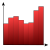Games
Problems
Go Pro!

# Pythagorean Means

Reference > Mathematics > StatisticsWhat is the arithmetic mean, and when should I use it? A brief statistics unitHarmonic Mean - what is it, and when should I use it?Geometric mean - what is the geometric mean, and when do we use it?Like us on Facebook to get updates about new resources